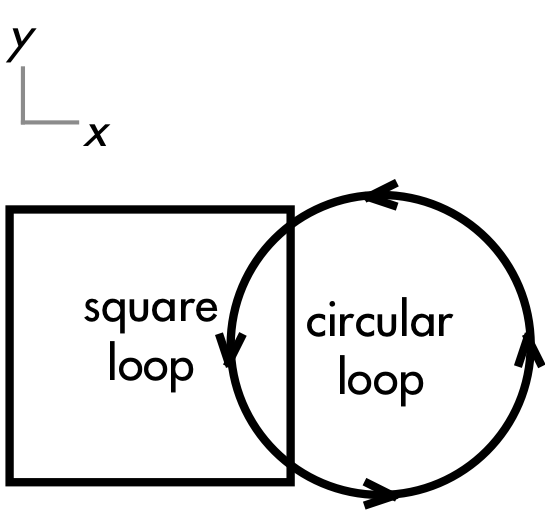## 20170507

### Physics midterm question: moving loop inducing current in stationary loop

Physics 205B Midterm 2, spring semester 2017
Cuesta College, San Luis Obispo, CAA moveable circular metal loop with a constant counterclockwise current (from an ideal emf source, not shown) partially overlaps a stationary square metal loop of resistance R. In order to create a counterclockwise induced current in the square loop, discuss whether the circular loop should be moved to the left or moved to the right (or if it is not possible to induce a counterclockwise current in the square loop). Explain your reasoning using the properties of magnetic fields, forces, motional emf, Faraday's law and Lenz's law.

• p:
Correct. Explains how a counterclockwise current can be induced in the square loop by moving the circular loop to the right (and/or the square loop to the left) by discussing:
1. the counterclockwise current in the circular loop creates a magnetic field that points out of the page everywhere within it, creating a magnetic flux that points out of the page in the overlapping region of the two loops; and
2. moving the circular loop to the right means that the overlapping portion of the two loops will decrease in area, decreasing the outwards magnetic flux; and
3. from Lenz's law this change in magnetic flux will through the square loop will be "fought" by an increasing outwards magnetic flux created by a counterclockwise current induced in the square loop.
• (May also or instead discuss the weaker magnetic field and flux outside of the circular loop, and/or using RHR1 to show that fictitious positive charges in the right-hand side of the square loop will experience an upwards force exerted by the circular loop's magnetic field as the circular loop moves to the right (equivalently meaning that the square loop would be moving to the left, relative to the circular loop).
• r:
As (p), but argument indirectly, weakly, or only by definition supports the statement to be proven, or has minor inconsistencies or loopholes.
• t:
Nearly correct, but argument has conceptual errors, or is incomplete. At least recognizes direction of the circular loop's magnetic flux, but misapplies Lenz's law to determine direction of induced current in the square loop and/or direction that the circular loop must be moved.
• v:
Limited relevant discussion of supporting evidence of at least some merit, but in an inconsistent or unclear manner. Some attempt at applying properties of magnetic fields, forces, motional emf, Faraday's law and Lenz's law. May say that this (static) outwards flux could be counteracted with the square loop's inwards flux, corresponding to a clockwise current, such that a counterclockwise current is not possible.
• x:
Implementation of ideas, but credit given for effort rather than merit. Approach other than that of applying properties of magnetic fields, forces, motional emf, Faraday's law and Lenz's law.
• y:
Irrelevant discussion/effectively blank.
• z:
Blank.
Sections 30882, 30883
Exam code: midterm02GruT
p: 4 students
r: 5 student
t: 10 students
v: 7 students
x: 3 students
y: 0 students
z: 0 students

A sample "p" response (from student 1921):Another sample "p" response (from student 7117):